Courses

# NCERT Exemplars - Communication Systems (Part - 1) Notes | EduRev

## Class 12 : NCERT Exemplars - Communication Systems (Part - 1) Notes | EduRev

The document NCERT Exemplars - Communication Systems (Part - 1) Notes | EduRev is a part of the Class 12 Course Physics Class 12.
All you need of Class 12 at this link: Class 12

MULTIPLE CHOICE QUESTIONS I

Q.1. Three waves A, B and C of frequencies 1600 kHz, 5 MHz and 60 MHz, respectively are to be transmitted from one place to another. Which of the following is the most appropriate mode of communication:
(a) A is transmitted via space wave while B and C are transmitted via sky wave
(b) A is transmitted via ground wave, B via sky wave and C via space wave
(c) B and C are transmitted via ground wave while A is transmitted via sky wave
(d) B is transmitted via ground wave while A and C are transmitted via space wave
Ans. (b)
Solution.
Key concept: The radio waves emitted from a transmitter antenna can reach the receiver antenna by the following mode of operation.

• Ground wave propagation
• Sky wave propagation
• Space wave propagation

Mode of communication frequency range:

• Ground wave propagation— 500 kHz to 1710 kHz
• Sky wave propagation — 2 MHz to 40 MHz
• Space wave propagation— 54 MHz to 42 GHz

So, A is transmitted via ground wave, B via sky wave and C via space wave.

Q.2. A 100m long antenna is mounted on a 500m tall building. The complex can become a transmission tower for waves with λ
(a) ~ 400 m
(b) ~ 25 m
(c) ~ 150 m
(d) ~ 2400 m
Ans.
(a)
Solution.
(a) Length of the building (l) is
l = 500 m
and length of antenna = 100 m
and we know, wavelength of the wave which can be transmitted by
L = λ/4.
So, λ~  4l = 4 x 100 = 400 m
Wavelength (λ) is nearly equal to 400 m.

Q.3. A 1 KW signal is transmitted using a communication channel which provides attenuation at the rate of – 2dB per km. If the communication channel has a total length of 5 km, the power of the signal received is [gain in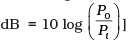(a) 900 W
(b) 100 W
(c) 990 W
(d) 1010 W
Ans.
(b)
Solution.
P= 1 kW = 1000 W
Rate of attenuation of signal = -2dB/km
Length of total path = 5km
Gain in attenuation = 5(-2) dB = -10dB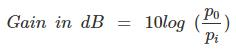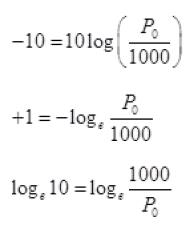Taking antilog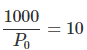P= 100 Watt

Q.4. A speech signal of 3 kHz is used to modulate a carrier signal of frequency 1 MHz, using amplitude modulation. The frequencies of the side bands will be
(a) 1.003 MHz and 0.997 MHz
(b) 3001 kHz and 2997 kHz
(c) 1003 kHz and 1000 kHz
(d) 1 MHz and 0.997 MHz
Ans.
(a)
Solution.
The process of changing the amplitude of a carrier wave in accordance with the amplitude of the audio frequency (AF) signal is known as amplitude modulation (AM).
In AM, frequency of the carrier wave remains unchanged.
Side band frequencies: The AM wave contains three frequencies fc, (fc + fm) and (fc - fm), fc is called carrier frequency, (fc + fm) and (fc - fm) are called side band frequencies.
(fc +fm): Upper side band (USB) frequency
(fc -fm): Lower side band (LSB) frequency
Side band frequencies are generally close to the carrier frequency.
According to the problem, frequency of carrier signal is fc = 1 MHz and frequency of speech signal = 3 kHz
= 3 x 10-3 MHz
= 0.003 MHz
We know that, Frequencies of side bands = (fc ± fm)
= (1 + 0.003) and (1 – 0.003)
So, side band frequencies are 1.003 MHz and 0.997 MHz.

Q.5.  A message signal of frequency ωm is superposed on a carrier wave of frequency ωc to get an amplitude modulated wave (AM).
The frequency of the AM wave will be
(a) ωm
(b) ωc
(c)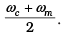(d)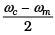Ans.
(b)
Solution.
The process of changing the amplitude of a carrier wave in accordance with the amplitude of the audio frequency (AF) signal is known as amplitude modulation (AM).
In AM, frequency of the carrier wave remains unchanged or we can say that the frequency of modulated wave is equal to the frequency of carrier wave. Now, according to the problem, frequency of carrier wave is fc.
Thus the amplitude modulated wave also has frequency fc.

Q.6. I-V characteristics of four devices are shown in Figure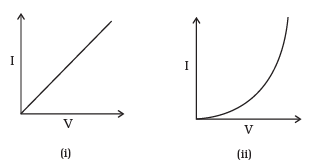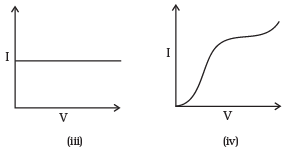Identify devices that can be used for modulation:
(a) i and iii
(b) Only iii
(c) ii and some regions of iv
(d) All the devices can be used

Ans. (c)
Solution.
A square law modulator is the device which can produce modulated waves by the application of the message signal and the carrier wave.
Square law modulator is used for modulation purpose. Characteristics shown by (i) and (iii) correspond to linear devices.
And by (ii) corresponds to square law device which shows non-linear relations. Some part of (iv) also follow square law.
Hence, (ii) and (iv) can be used for modulation.

Q.7. A male voice after modulation-transmission sounds like that of a female to the receiver. The problem is due to
(a) Poor selection of  modulation index (selected 0 < m < 1)
(b) Poor bandwidth selection of amplifiers
(c) Poor selection of carrier frequency
(d) Loss of energy in transmission
Ans.
(b)
Solution
In this problem, the frequency of modulated signal received becomes more, due to improper selection of bandwidth.
This happens because bandwidth in amplitude modulation is equal to twice the frequency of modulating signal.
But, the frequency of male voice is less than that of a female.

Q.8. A basic communication system consists of
(A) transmitter
(B) information source
(C) user of information
(D) channel
Choose the correct sequence in which these are arranged in a basic communication system:
(a) ABCDE
(c) BDACE
Ans.
(b)
Solution.
A basic communication system consists of an information source, a transmitter, a link (channel) and a receiver or a communication system is the set-up used in the transmission and reception of information from one place to another.
The whole system consist of several elements in a sequence. It can be represented as the diagram given below: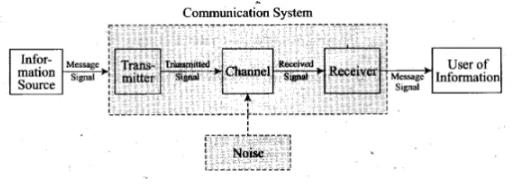Q.9. Identify the mathematical expression for amplitude modulated wave:
(a) Ac sin [{ωc + k1vm(t )}t + φ ]
(b) Ac sin {ωct + φ + k2 vm(t)}
(c) {Ac + k2 vm(t)} sin (ωct + φ )
(d) Ac vm(t) sin (ωct +  φ )
Ans.
(c)
Solution.
Consider a signal to be modulated is m(t) =Am sin ωmt where Am is amplitude of modulated signal
Am= Ac + m(t)
A=Ac + Am sin ωmt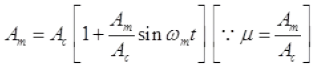ωm = 2πvmφvm (Vm = frequency of signal)
C(t) =Ac sinωe t
Cm(t) =C(t) +m(t)
Cm(t)=Am sin ωct
Cm(t)= Ac[1+μ sin ωmt] sin ωct
= {Ac + μAc  sin ωmt} sin (ωct +φ ) (φ = phase diff.)
Let μAc = k2
Sin ωmt= vm(t)
∴ Cm(t)={Ac+k2  vm  (t)} sinφ )

Multiple Choice Questions II

Q.10. An audio signal of 15 kHz frequency cannot be transmitted over long distances without modulation because
(a) The size of the required antenna would be at least 5 km which is not  convenient.
(b) The audio signal can not be transmitted through sky waves.
(c) The size of the required antenna would be at least 20 km, which is not convenient.
(d) Effective power transmitted would be very low, if the size of the antenna is less than 5 km
Ans. (a,b,d)
Solution.
Frequency of audio signal vm=15 kHZ

=15 × 103 Hz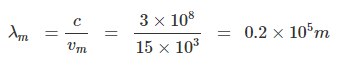Size of antenna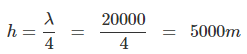Size of antenna= 5 km
Audio frequency signal are of low frequency waves. So the audio wave cannot be transmitted by sky wave as they are absorbed by atmosphere. If the size of the antenna is less than 5 km, the effective power transmission would be very low because of the deviation from resonance of wavelength of wave and antenna length.

Q.11. Audio sine waves of 3 kHz frequency are used to amplitude modulate a carrier signal of 1.5 MHz. Which of the following statements are true?
(a) The side band frequencies are 1506 kHz and 1494 kHz
(b) The bandwidth required for amplitude modulation is 6 kHz
(c) The bandwidth required for amplitude modulation is 3 MHz
(d) The side band frequencies are 1503 kHz and 1497 kHz
Ans.
(b,d)
Solution.
Side band frequencies: The AM wave contains three frequencies fc, (fc + fm) and (fc -fm), fc  is called carrier frequency. (fc +fm) and (f- fm) are called side band frequencies.
(fc +fm) Upper side band (USB) frequency
(f- fm) Lower side band (LSB) frequency
Side band frequencies are generally close to the carrier frequency.
According to the problem, frequency of audio sine wave fm = 3 kHz and frequency of earner signal fc = 1.5 MHz = 1500 kHz
Now, side band frequencies
f± f= (I500 ± 3)
= 1503 kHz and 1497 kHz
USB = 1503 kHz and LSB = 1497 kHz
Also,bandwidth = 2 f= 2 x 3 = 6 KHz

Q.12 A TV transmission tower has a height of 240 m. Signals broadcast from this tower will be received by LOS communication at a distance of (assume the radius of earth to be 6.4 × 106 m)
(a) 100 km
(b) 24 km
(c) 55 km
(d) 50 km
Ans.
(b,c,d)
Solution.
h= 240 m R = 6.4 × 10m
For LOS communication range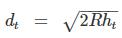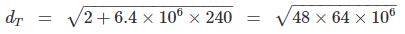=4 x 8 x 103√3 = 32000 x 1.732 m
= 55.424 km = 55424 m
So, the range 55.424 km covers the range 24 km, 55 km and 50 km.

Q.13. The frequency response curve (Figure) for the filter circuit used for production of AM wave should be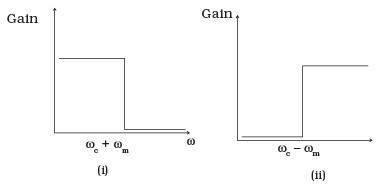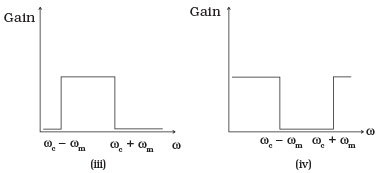(a) (i) followed by (ii)
(b) (ii) followed by (i)
(c) (iii)
(d) (iv)

Ans. (a,b,c)
Solution.
production of amplitude modulated wave bandwidth is given by equal to
Frequency of upper side band- frequency lower side band Bandwidth= (ωcm)-(ωcm)=2ωm

Q.14. In amplitude modulation, the modulation index m , is kept less than or equal to 1 because
(a) m > 1, will result in interference between carrier frequency and message frequency, resulting into distortion.
(b) m > 1 will result in overlapping of both side bands resulting into loss of information. (c) m > 1 will result in change in phase between carrier signal and message signal.
(d) m > 1 indicates amplitude of message signal greater than amplitude of carrier signal resulting into distortion
Ans.

Solution.
modulation index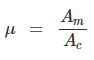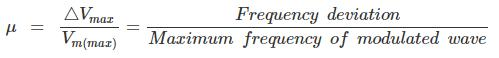If mf > 1 then Δvmax > vm . This means there will be overlapping of both the side bands of modulated wave resulting into loss of information.

Q.15. Which of the following would produce analog signals and which would produce digital signals?
(i) A vibrating tuning fork.
(ii) Musical sound due to a vibrating sitar string.
(iii) Light pulse.
(iv) Output of NAND gate

Ans. Analog and digital signals are the gateway of information or we can say that they are used to transmit information through electric signals. In both these signals, the
information such as any audio or video is transformed into electric signals.
The difference between analog and digital technologies is that in analog technology, information is translated into electric pulses of varying amplitude. In digital technology, translation of information is into binary formal (zero or one) where each bit is representative of two distinct amplitudes. So, output of a NAND gate and a light pulse produces a digital signal.
Thus, (a) and (b) would produce analog signal and (c) and (d) would produce digital signals.

Q.16. Would sky waves be suitable for transmission of TV signals of 60 MHz frequency?
Ans.
A signal of frequency range 1710 kHz to 40 MHz can be transmitted by sky waves.
Frequency of T.V. signals are 60 MHz which is beyond the sky wave so, T.V. transmission cannot be made by sky wave transmission. Given signal can be transmitted by space wave transmission.

Q.17. Two waves A and B of frequencies 2 MHz and 3 MHz, respectively are beamed in the same direction for communication via sky wave. Which one of these is likely to travel longer distance in the ionosphere before suffering total internal reflection?
Ans.
As the frequency of wave B is more than A and μ α v so refractive index for wave B will be more than A.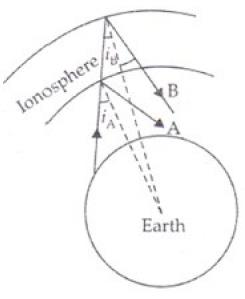Critical angle is inversely proportional to refractive index (sin ic = 1/μ). So critical angle for A will be larger than for B or in other way
vA < vB [Given]
μA < μB
[∴ μ ∝ v (frequency)]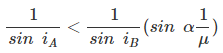Sin iA > sin iB (sin i α i)
iA > iB
It is clear from figure also that ray B will travel more distance in ionosphere before getting total internal reflection through ionosphere.

Q.18. The maximum amplitude of an A.M. wave is found to be 15 V while its minimum amplitude is found to be 3 V. What is the modulation index?
Ans.
Maximum amplitude of A.M. wave = Amax
Minimum amplitude of A.M. wave = Amin
Amax =Ac+A= 15V …….(I)
Amin= Ac-A= 3 V ……(II)
Where Ac = amplitude of carrier wave, Am= amplitude of A.M. wave
Ac = 9 V
Am = 6 V (From I and II)
∴ Modulation index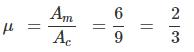Q.19. Compute the LC product of a tuned amplifier circuit required to generate a carrier wave of 1 MHz for amplitude modulation.
Ans. Frequency of tuned amplifier or resonance frequency is given by v =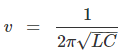which generate a carrier wave of I MHz for amplitude modulation
So, we get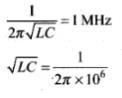Squaring both sides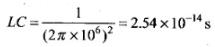Thus, the product of LC is 2.54 x 10-14s.

Q.20. Why is a AM signal likely to be more noisy than a FM signal upon transmission through a channel?
Ans.
An AM signal likely to be more noisy than FM signal through a channel because in case of AM, the instantaneous voltage of carrier waver waves is varied by the modulating wave voltage So, during the transmission, noise signals can also be added and receiver assumes noise a part of the modulating signal. In case of FM, the frequency of carrier waves is changed as the change in the instantaneous voltage of modulating waves. This can be done by mixing and not while the signal transmitting in channel. So, noise does not affect FM signal or simply we can say that noise signals are difficult to filter out in AM reception whereas FM receivers easily filter out noise.
Important point: In frequency modulation mf (frequency modulation index) is inversely proportional to modulating frequency fm. While in PM it does not vary with modulating frequency. Moreover, FM is more noise immune.

Offer running on EduRev: Apply code STAYHOME200 to get INR 200 off on our premium plan EduRev Infinity!

,

,

,

,

,

,

,

,

,

,

,

,

,

,

,

,

,

,

,

,

,

;